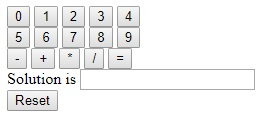#Easiest HTML Calculator Eample CodeThere are plenty of ways to do math on a desktop computer using a built-in calculator, but another way is to build one yourself using a simple HTML code. To create a calculator using HTML, learn some basics about HTML, then copy the necessary code into a text editor and save it with an HTML extension. You can then use your calculator by opening up the HTML document in your favorite browser. By doing all of this, not only will you be able to do math in a browser, but you can also learn some fundamentals about the art of coding!

1. Copy the code below. Highlight the text in the box below by holding down your cursor in the top-left corner of the box, and dragging it to the bottom-right corner of the box so that all of the text is blue. Then, press "Command+C" on a Mac or "Ctrl+C" on a PC to copy the code the clipboard.

```<html>
<title>HTML Calculator</title>
<body bgcolor= "#000000" text= "gold">
<form name="calculator" >
<input type="button" value="0" onClick="document.calculator.ans.value+='0'">
<input type="button" value="1" onClick="document.calculator.ans.value+='1'">
<input type="button" value="2" onClick="document.calculator.ans.value+='2'">
<input type="button" value="3" onClick="document.calculator.ans.value+='3'">
<input type="button" value="4" onClick="document.calculator.ans.value+='4'"><br />
<input type="button" value="5" onClick="document.calculator.ans.value+='5'">
<input type="button" value="6" onClick="document.calculator.ans.value+='6'">
<input type="button" value="7" onClick="document.calculator.ans.value+='7'">
<input type="button" value="8" onClick="document.calculator.ans.value+='8'">
<input type="button" value="9" onClick="document.calculator.ans.value+='9'"><br />
<input type="button" value="-" onClick="document.calculator.ans.value+='-'">
<input type="button" value="+" onClick="document.calculator.ans.value+='+'">
<input type="button" value="*" onClick="document.calculator.ans.value+='*'">
<input type="button" value="/" onClick="document.calculator.ans.value+='/'">
<input type="button" value="=" onClick="document.calculator.ans.value=eval(document.calculator.ans.value)"><br />
Solution is <input type="textfield" name="ans" value=""><br />
<input type="reset" value="Reset">
</form>
</body>
</html>
```

2. Open a text editing program on your computer. There are a number of programs you can use, but for convenience and quality, we recommend using TextEdit or Notepad.

On a Mac, click on the magnifying glass at the top-right corner of your screen to open Spotlight. Once there, type in TextEdit and click on the TextEdit program, which should now be highlighted in blue.

On a PC, open the Start menu at the bottom-left corner of your screen. In the search bar, type Notepad and click on the Notepad application, which will appear in the results bar to the right.

3. Paste the HTML code for a calculator into the document.

On a Mac, click on the body of the document and press "Command+V". You will then need to click on "Format" at the top of your screen and click "Make Plain Text" after pasting the code.

On a PC, click on the body of the document and press "Ctrl+V".

4. Understand what each html function is doing. The code you will use to create your calculator is made up of many pieces of syntax that work together to define different elements of a document. Click here for an explanation of how to familiarize yourself with this process, or read on to learn what each line of text is doing in the code you'll be using to make your calculator.

html: This piece of syntax tells the rest of the document what language is being used in the code. In coding, a number of languages used to code, and <html> tells the rest of the document that it will be in - you guessed it! - html.

head: Tells the document that everything beneath it is data about data, also known as "metadata". The <head> tag is usually used to define stylistic elements of a document, such as titles, headings, and so on. Think of it as an umbrella under which the rest of the code is defined.

title: This is where you will name the title of your document. This attribute is used to define what the title of the document will be when opened in an html browser.

body bgcolor= "#": This attribute sets the color of the code's background and body. The number in this set of quotes that appears after # corresponds to a predetermined color.

text= "": The word in this set of quotes sets the color of the text on the document.

form name="": This attribute specifies the name of a form, which is used to build the structure of what comes after it based on what Javascript knows that form name to mean. For example, the form name we will be using is calculator, which will create a specific structure to the document.

input type="": This is where the action happens. The "input type" attribute tells the document what sort of text the values in the rest of the brackets are. For example, they could be text, a password, a button (as it will be for a calculator), and so on.

value="": This command tells the document what will be contained in the input type specified above. For a calculator, these show up as our numbers (1-9) and operations (+,-,*,/,=).

onClick="": This syntax describes an event, which tells the document that something should occur when the button is clicked. For a calculator, we want the text that displays in each button to by understood as such. So, for the "6" button, we'll put document.calculator.ans.value+='6' between the quotes.

br: this tag initiates a line break in the document, so that whatever comes after it will appear a line below whatever came before it.

/form, /body, and /html: these commands tell the document that the corresponding commands that were initiated earlier in the document are now ending.

5. Save the file. To do this, click on the "File" button at the top-left hand of your window, and click on "Save As..." on a PC or "Save..." on a Mac in the menu that drops down.

6. Add an HTML extension to the file name. In the "Save As..." menu, type in your file name followed by ".html", and then click "Save". For example, if you wanted to call this file my first calculator, you would save the file as "MyFirstCalculator.html"